# NOIp算法（一）—— 快速幂

NOIp结束了，我退役了。

$latex \large a^{b} = \begin{matrix}\underbrace{a\times a\times \cdots\times a}\\b\end{matrix}$

$latex \large 11=1*2^{3} + 0*2^{2}+ 1*2^{1} + 1*2^{0}$

$latex \large a^{11}=a^{1*2^{3} + 0*2^{2}+ 1*2^{1} + 1*2^{0}}=a^{2^{3}}*a^{2^{1}}*a^{2^{0}}$

$latex \large \begin{matrix}a^{2^3}&a^{2^2}&a^{2^1}&a^{2^0}\\1&0&1&1\end{matrix}$

$latex \large a^{2^{n-1}}$

so,

long long qpow(long long a,long long b){\\快速幂
long long ans = 1;//ans为要输出的答案
long long base = a;//因为我们先要算第1位，所以先赋值base为a^(2^0)，
while(b != 0){//如果b已经移位成0，也就是每一位都处理完，就是算完了
if(b % 2 == 1){//如果b的二进制最后一位是1的话
ans *= base;//把答案乘以 a^(2^(n-1))
}
base *= base;//每一次循环自乘一次，这样保证base就为a^(2^(循环次数-1))，也就是a^(2^(n-1))
b >>= 1;//把b右移一位，这样下次循环b的最后一位就是第二位
}
return ans;
}

$latex \large b\&1$

long long qpow(long long a,long long b){long long ans = 1, base = a;while(b){if(b & 1) ans *= base;//判断b的二进制最后一位是否为1base *= base;b >>= 1;}return ans;}

1.缪雪儿.佛兰说道：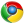Google Chrome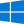Windows 10
唔姆，实用
2.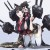Arbiter丶说道：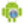WebView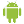Android 7.1.1
唔姆，不会用。
3.Arbiter丶说道：WebViewAndroid 7.1.1
唔姆，不会用。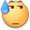4.余音歆风说道：Google ChromeWindows 10
啊这……这不是我为了提高程序效率找了半天的那个算法吗……